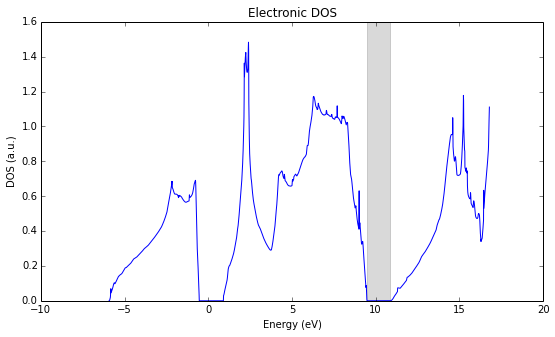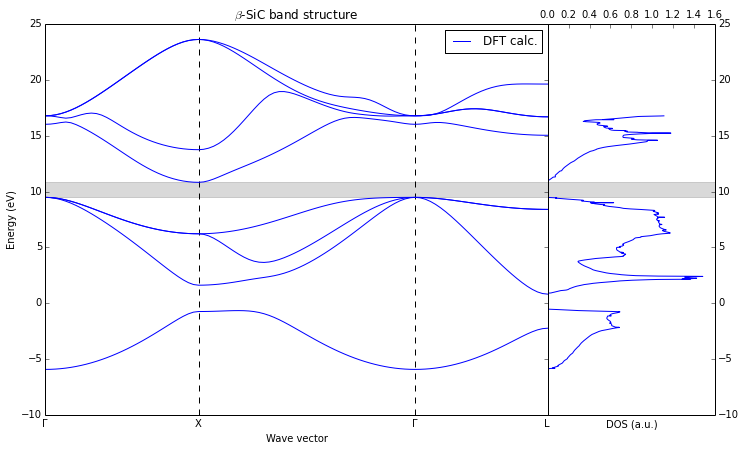# Electronic structure calculations¶

This is a part in the tutorial series. Read the previous tutorials to understand all material presented here. The initial setup and other issues described in earlier parts will not be repeated.

Electronic structure is a fundamental property of any crystal. The determination of the electronic structure is a first step in any quantum mechanical calculation. The description of the methods used by the Quantum Espresso package and the DFT approach are discussed in many review papers and textbooks (e.g. M. Payne). Review of this paper or some other handbook on DFT calculations is highly recommended before starting any serious computational projects.

To continue skip over the initial setup to the second cell below.

In :
# Import the basic libraries

# ASE system
import ase
from ase import Atom, Atoms
from ase import io
from ase.lattice.spacegroup import crystal
from __future__ import division

# Spacegroup/symmetry library
from pyspglib import spglib

# iPython utility function
from IPython.core.display import Image

# Import the tools from the qe-util package
from qeutil import RemoteQE
from qeutil.analyzers import plot_bands

# Access info
import host


## Preparations¶

To start any calculation we need to create a crystal and the calculator (see earlier tutorials for details). We will use a cubic, zinc-blende (F-43m) SiC crystal again, with a lattice constant determined in our first tutorial.

The Quantum Espresso calculator will use a denser Brillouin zone grid (kpts: 8x8x8) than in previous examples to better reproduce and sample the electronic configuration in the crystal.

In :
qe=RemoteQE(label='SiC-elec',   # A name for the project
kpts=[8,8,8],    # Dense k-space grid
xc='pz',         # Exchange functional type in the name of the pseudopotentials
pp_type='vbc',   # Variant of the pseudopotential
pp_format='UPF', # Format of the pseudopotential files
ecutwfc=70,      # Energy cut-off
pseudo_dir='../../pspot',
use_symmetry=True,
procs=8)         # Use 8 cores for the calculation

print qe.directory

calc/SiC-elec.Quqnj_

In :
a=4.3366
cryst = crystal(['Si', 'C'],
[(0, 0, 0), (0.25, 0.25, 0.25)],
spacegroup=216,
cellpar=[a, a, a, 90, 90, 90])

# Assign the calculator to our system
cryst.set_calculator(qe)


## Structure check¶

Run the calculation to get stress tensor (Voigt notation in GPa), to make sure our structure is reasonably close to the equilibrium lattice constant.

Note: The change in k-space sampling influenced the equilibrium lattice constant, and the stress is not close to zero any more. In fact the equilibrium lattice constant for a 8x8x8 k-space sampling is A=4.3341 A. To keep the structure at P=0 we change the lattice constant to the appropriate value. Note how the calculation was automatically re-done after we have changed the lattice constant. The scale_atoms=True makes all atoms move with the unit cell - otherwise the positions of atoms in Cartesian coordinates will stay the same and we would destroy the structure!

In :
print "Stress tensor before and after change (Voigt notation, GPa):\n", cryst.get_stress()/ase.units.GPa

a=4.3341
cryst.set_cell([a,a,a],scale_atoms=True)

print cryst.get_stress()/ase.units.GPa

Stress tensor before and after change (Voigt notation, GPa):
[ 0.381  0.381  0.381  0.    -0.    -0.   ]
[-0.007 -0.007 -0.007  0.    -0.    -0.   ]


## Electronic structure¶

The electronic structure calculation provides two main results:

• The band structure
• Density of states function

The band structure is calculated along some path in the Brillouin zone which must be specified before the start of the calculation. It is specified with the parameter qpath to the calculator. The points parameter specifies how many data points should be calculated along the path. For the DOS calculation we need to specify the grid for the reciprocal space integration (nkdos). Additionally we need to specify number of bands included in the calculation (nbnd parameter), since otherwise the unoccupied levels will not be included. If we want to obtain information about band gap and the shape of excited levels we need to include them in the calculation. The default value for the insulator is equal to number of valence bands (number of electrons/2) and for metal it is larger by 20% (minimum 4 levels).

The reciprocal space points are specified in Cartesian coordinates in units of $2\pi/a$ (the same rule as for the phonons). You can find the coordinates of special points in the Brillouin zone using the Bilbao Crystallographic Server (the correct coordinates are in the "Conventional basis" column of the table.)

In :
# Several special points in the FCC lattice
G1=[0,0,0]
G2=[1,1,1]
X=[0,1,0]
L=[1/2,1/2,1/2]
W=[1/2,1/4,3/4]
K=[sqrt(1/2),sqrt(1/2),0]
M=[1,1,0]

# Set the path along which we would like to plot the electronic structure
qpath=array([G1,X,G2,L])

# Name the special points for plotting
qpname=[u'Γ','X',u'Γ','L']

qe.set(nbnd=8)                 # Increase the number of bands to include excited states
qe.set(qpath=qpath)            # Define the path for the band structure calculation
qe.set(points=100)             # Set number of points along the path
qe.set(nkdos=[15,15,15])       # Set the grid for reciprocal space integration of states

# execute the calculation of band structure and electronic dos
qe.calculate(cryst,properties=['bands','edos'])


## Analysing the results¶

After the calculation the results are imported into the results dictionary of the calculator and can be easily for analysis. Let us first check the band gap in the crystal. The Highest Occupied Level (HOL) and Lowest Unoccupied Level (LUL) entries in the results dictionary contain energies of the lower and upper limit of the band gap.

It is a well-known fact that the DFT procedure strongly underestimates the band gap in most of the materials. Thus it is not surprising that the result we have obtained is also substantially lower then the experimental value (2.36 eV).

In :
# Print the position and size of the band gap
print "Highest Ocupied Level  (HOL): %(HOL)8.2f eV \nLowest Unocupied Level (LUL): %(LUL)8.2f eV" % qe.results
print "Band gap: %.2f eV" % (qe.results['LUL'] - qe.results['HOL'])

Highest Ocupied Level  (HOL):     9.48 eV
Lowest Unocupied Level (LUL):    10.83 eV
Band gap: 1.35 eV


The Density of States (DOS) function is imported into the edos entry of the results dictionary and can be easily plotted and further analyzed. Here, we will plot the function and mark the band gap with a gray band.

Note: The Fermi energy level (HOL in the insulator at T=0K) is not at zero. The absolute value of energy has no physical meaning and the Quantum Espresso programs do not move this energy to zero - as is sometimes customary.

In :
figsize(9,5)
plot(qe.results['edos'],qe.results['edos'])
axvspan(qe.results['HOL'], qe.results['LUL'], alpha=0.15, color='k'); # Mark the band gap with the gray rectangle
title('Electronic DOS')
xlabel('Energy (eV)')
ylabel('DOS (a.u.)');## Band structure¶

The band structure can be plotted "by hand" easily enough but doing it in this way is fairly involved. The results are imported into the bands and bands_kpt entries of the dictionary. However, the QE-util library provides a utility function for creating a "standard" band structure plot. The function needs on input the results dictionary from the calculation as well as specification of the special points along the path as well as their names. The return value of the function is a pair of handles to sub-plots of the figure, which provides the simple way to further customize the figure (as is shown below).

In :
figsize(12,7)
ax1,ax2=plot_bands(qe.results, qpath=qpath, qpname=qpname, label='DFT calc.')
ax2.legend();
ax2.set_xlabel('Wave vector')
ax2.set_ylabel('Energy (eV)')
ax2.set_title('$\\beta$-SiC band structure')
ax1.set_xlabel('DOS (a.u.)');In :